If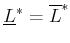, the security strategies are called a saddle point, and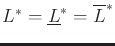is called the value of the game. If this occurs, the order of the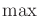and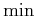can be swapped without changing the value: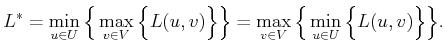(9.51)

A saddle point is sometimes referred to as an equilibrium because the players have no incentive to change their choices (because there is no regret). A saddle point is defined as any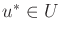and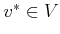such that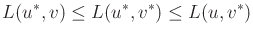(9.52)

for all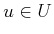and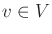. Note that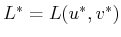. When looking at a matrix game, a saddle point is found by finding the simple pattern shown in Figure 9.2.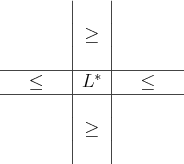Example 9..14 (A Deterministic Saddle Point)   Here is a matrix game that has a saddle point: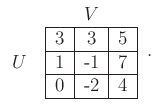(9.53)

By applying (9.52) (or using Figure 9.2), the saddle point is obtained when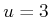and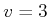. The result is that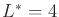. In this case, neither player has regret after the game is finished.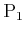is satisfied because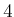is the lowest cost it could have received, given that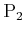chose the third column. Likewise,is the highest cost thatcould have received, given thatchose the bottom row.What if there are multiple saddle points in the same game? This may appear to be a problem because the players have no way to coordinate their decisions. What iftries to achieve one saddle point whiletries to achieve another? It turns out that if there is more than one saddle point, then there must at least be four, as shown in Figure 9.3. As soon as we try to make two +'' patterns like the one shown in Figure 9.2, they intersect, and four saddle points are created. Similar behavior occurs as more saddle points are added.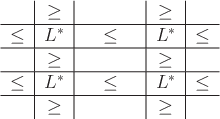Example 9..15 (Multiple Saddle Points)   This game has multiple saddle points and follows the pattern in Figure 9.3: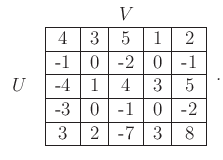(9.54)

Let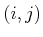denote the pair of choices forand, respectively. Both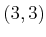and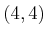are saddle points with value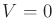. What ifchooses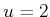andchooses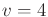? This is not a problem because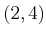is also a saddle point. Likewise,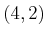is another saddle point. In general, no problems are caused by the existence of multiple saddle points because the resulting cost is independent of which saddle point is attempted by each player.Steven M LaValle 2020-08-14